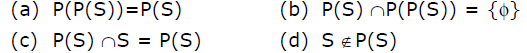# GATE | GATE-CS-2000 | Question 29

Let P(S) denotes the power set of set S. Which of the following is always true?(A) a
(B) b
(C) c
(D) d

Explanation:

Ex:  let a set ‘s’ be, s={1,2}

Power-set(s)=P(s)={{},{1},{2},{1,2}} Note: ‘{}’ denotes empty or NULL set or φ.

In general, if a set ‘s’ contains ‘n’ elements,  power-set will contain 2^n elements, that denotes set of all subset of ‘s’.
Solution: Option (a): P(P(S))=P(S) If, S={1} P(S)={ φ, {1}} P(P(S))={ φ,{ φ},{1},{ φ, {1}}}

NOTE: LHS is set of set of subsets of ‘S’ and RHS is set of subsets of ‘S’. So, we can conclude this option is false.
Option (b): P(P(S)) ∩P(S)={ φ}.

If, S={1}
P(S)={ φ, {1}}
P(P(S))={ φ,{ φ},{1},{ φ, {1}}}
P(P(S)) ∩P(S)= { φ}=RHS
So, we can conclude this option is TRUE.

Option (a): P(S) ∩S=P(S)
If, S={1}
P(S)={ φ, {1}}
P(S) ∩S= φ
NOTE: We can’t find anything common in set of elements (S) and set of sets(P(S)).
So, we can conclude this option is false.
Option (a): S ∉ P(S)
If, S={1}
P(S)={ φ, {1}}
Clearly, S is subset of P(S).
NOTE: By definition only, its clear that power-set is set of all subset that will contain S also.
So, we can conclude this option is false.

This solution is contributed by Sandeep pandey.

Quiz of this Question

My Personal Notes arrow_drop_up
Article Tags :

Be the First to upvote.

Please write to us at contribute@geeksforgeeks.org to report any issue with the above content.